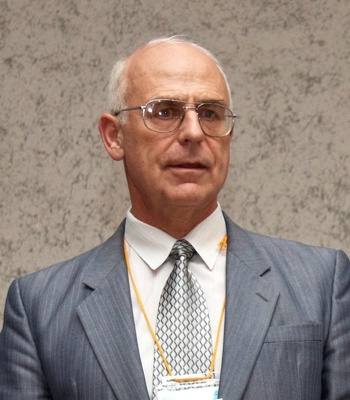S.M. Nikol’skii Mathematical Institute
S.M. Nikol’skii Mathematical Institute
S.M. Nikol’skii Mathematical Institute conducts research in the field of functional analysis, theory of functional spaces, ordinary differential equations, partial differential equations, nonlinear analysis, spectral theory of differential operators.Director
Alexander Skubachevskii
Contacts
News All news
Science
27 Jun
RUDN Mathematicians: 5G will cope with traffic when using WiGig

RUDN mathematicians investigated the possibility of combining 5 GNR technology and WiGig — a high-frequency range that allows you to transfer data at speeds up to 10 Gbps. This will smooth traffic fluctuations in 5Gnetworks and cope with user requests.

Science
23 Jun
Scientists Reveal the Water Column of the Yamal Lakes Can Be a Microbial Filter

Scientists from the Winogradsky Institute of Microbiology RAS, RUDN University, St. Petersburg State University and the Tyumen Scientific Centre SB RAS studied the microbial communities from several lakes of the Yamal Peninsula. It turned out that methanotrophs (bacteria that use methane as a source of energy) consume methane more actively in the deep mature lakes of the peninsula than in small thermokarst lakes. In this regard, methane emissions into the atmosphere from the surface of deep lakes are low, and only small (relatively younger thermokarst lakes with constitutional ground ice) can make a significant contribution to methane emissions in the north of Western Siberia. Thus, bacteria perform an important function for the climate balance — they reduce the emission of methane into the atmosphere.

Science
20 Jun
RUDN University Physicists Determine the Optimal Conditions for Holding High-Energy Plasma Clouds in Pyrotron

RUDN University physicists have described the conditions for the most efficient operation of long mirror-based variant of cyclotron in the autoresonance mode. These data will bring better understanding of plasma processes in magnetic traps.

Main areas of research All areas
Research projects All projects
The main idea of the project: research of new classes of differential and functional-differential equations, inequalities and systems and the application of the obtained results to interdisciplinary research in mathematical models of physical and biological processes.

Many problems that are studied in mathematical analysis can be reformulated in terms of the action of various operators in function spaces.

Construction of spherically symmetric stationary solutions of the Vlasov-Poisson system of equations describing the stationary distribution of particles in a gravitational field. Obtaining sufficient conditions for confining high-temperature plasma in a “mirror-trap” fusion reactor.

Laboratories and centers All laboratories

The center is a structural subdivision of the S.M. Nikol’skii Mathematical Institute specializes in the field of mathematical physics.

TypeCenter

Mathematical modelling in biomedicine is one of rapidly developing scientific disciplines motivated by the fundamental research and by the applications to public health.

TypeCenter

Partners All partners
Events All events
2022
24 May
Seminar “Boundary value problems for semilinear Schrodinger equations with Hardy-type potentials”
We consider a class of semi-linear elliptic equations involving a Hardy-type potential and an absorption nonlinear term.
2022
24 May
Scientific seminar “The second boundary value problem for differential-difference equations with variable coefficients on an interval of integer length”
We consider the second boundary value problem for a second-order differential-difference equation with a difference operator with variable coefficients on an interval of integer length.
2022
5 May
Seminar “Using reduced models to generate signals that resemble brain rhythms”
In this talk, I will discuss some models with the objective to generate signals that resemble brain rhythms.